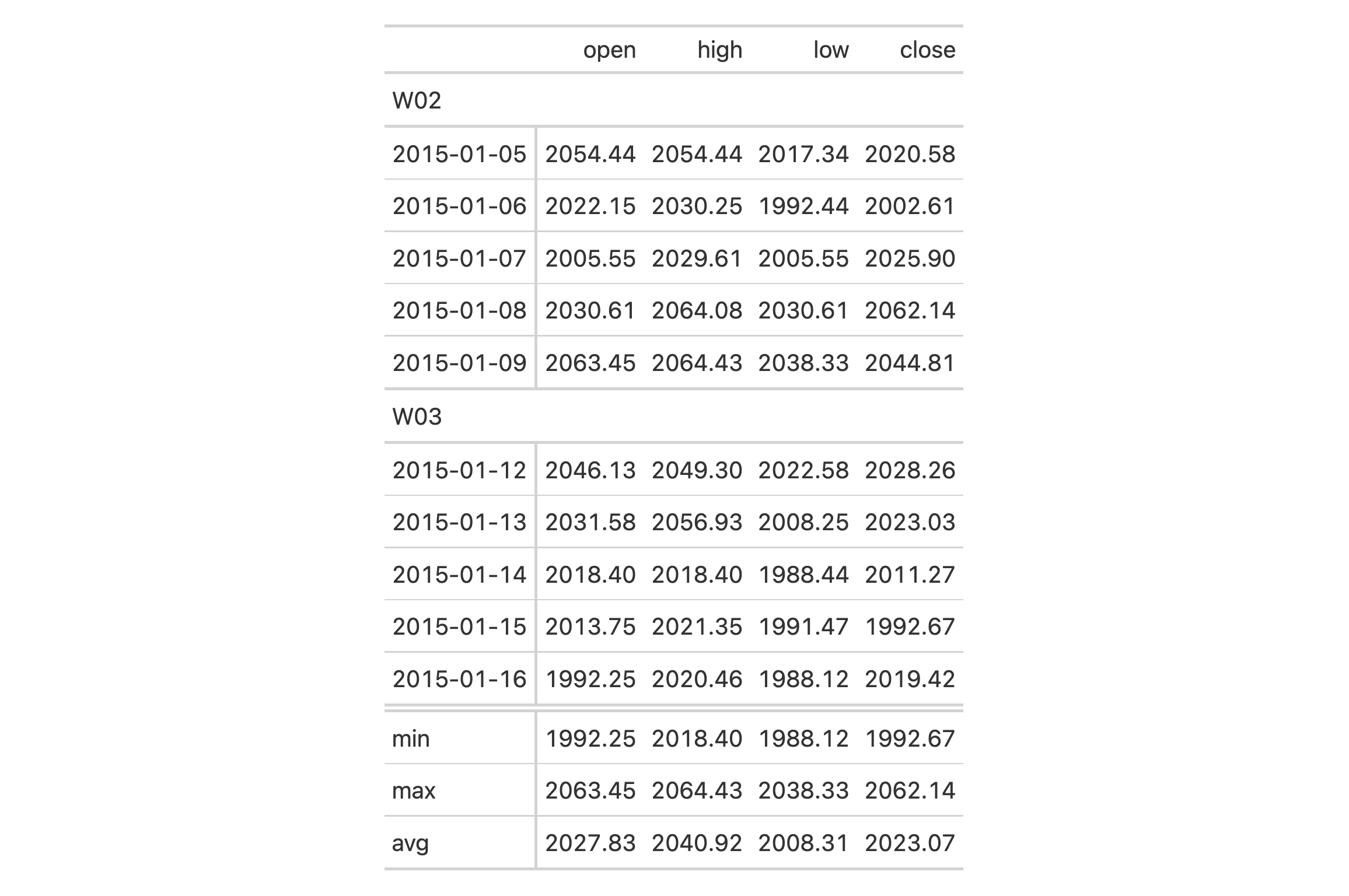# grand_summary_rows: Add grand summary rows using aggregation functions In gt: Easily Create Presentation-Ready Display Tables

 grand_summary_rows R Documentation

## Add grand summary rows using aggregation functions

### Description

Add grand summary rows to the gt table by using applying aggregation functions to the table data. The summary rows incorporate all of the available data, regardless of whether some of the data are part of row groups. You choose how to format the values in the resulting summary cells by use of a `formatter` function (e.g, `fmt_number`) and any relevant options.

### Usage

```grand_summary_rows(
data,
columns = everything(),
fns,
missing_text = "---",
formatter = fmt_number,
...
)
```

### Arguments

 `data` A table object that is created using the `gt()` function. `columns` The columns for which the summaries should be calculated. `fns` Functions used for aggregations. This can include base functions like `mean`, `min`, `max`, `median`, `sd`, or `sum` or any other user-defined aggregation function. The function(s) should be supplied within a `list()`. Within that list, we can specify the functions by use of function names in quotes (e.g., `"sum"`), as bare functions (e.g., `sum`), or as one-sided R formulas using a leading `~`. In the formula representation, a `.` serves as the data to be summarized (e.g., `sum(., na.rm = TRUE)`). The use of named arguments is recommended as the names will serve as summary row labels for the corresponding summary rows data (the labels can derived from the function names but only when not providing bare function names). `missing_text` The text to be used in place of `NA` values in summary cells with no data outputs. `formatter` A formatter function name. These can be any of the `fmt_*()` functions available in the package (e.g., `fmt_number()`, `fmt_percent()`, etc.), or a custom function using `fmt()`. The default function is `fmt_number()` and its options can be accessed through `...`. `...` Values passed to the `formatter` function, where the provided values are to be in the form of named vectors. For example, when using the default `formatter` function, `fmt_number()`, options such as `decimals`, `use_seps`, and `locale` can be used.

### Details

Should we need to obtain the summary data for external purposes, the `extract_summary()` function can be used with a `gt_tbl` object where grand summary rows were added via `grand_summary_rows()`.

### Value

An object of class `gt_tbl`.

### Examples

Use `sp500` to create a gt table with row groups. Create the grand summary rows `min`, `max`, and `avg` for the table with the `grand_summary_rows()` function.

```sp500 %>%
dplyr::filter(date >= "2015-01-05" & date <= "2015-01-16") %>%
dplyr::arrange(date) %>%
dplyr::mutate(week = paste0("W", strftime(date, format = "%V"))) %>%
gt(
rowname_col = "date",
groupname_col = "week"
) %>%
grand_summary_rows(
columns = c(open, high, low, close),
fns = list(
min = ~min(.),
max = ~max(.),
avg = ~mean(.)),
formatter = fmt_number,
use_seps = FALSE
)
```### Function ID

5-2

Other row addition/modification functions: `row_group_order()`, `summary_rows()`